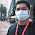## Friday, March 09, 2012

### Positioning and Mirroring Images in HTML5... ...and Dragons!

While working on our most recent game, Todd implored me to sharpen some of our sprite rendering. Fuzzy sprites are a result of antialiasing and in our case some images were undergoing this process twice. Rendering pixel to pixel makes a much sharper image, especially if the image has already been anti-aliased once. For All the King's Men, we check the screen size and automatically create all the art at the correct resolution by scaling our sprites from the original size to the appropriate size needed for the current resolution (determined in part by our canvas auto-resizing). Since we pre-render the art at the correct size, and anti-alias it in the process, the last thing we want to do is render it to the visible canvas with a pixel offset, effectively anti-aliasing it twice. Todd accuses me of implementing my awesome "Blurrification Technology" whenever he noticed me doing this. This is how I addressed it (and hopefully stopped him from using made-up words).

At right is a scaled dragon sprite that is still relatively clear, after it's initial anti-aliasing. Also note the sharp color bars I've inserted to highlight the blur. This image is rendered at (0,0), so each pixel on the destination canvas matches a pixel on the source canvas. If our source image was stored in img and the canvas context is ctx, the code might look like this:

```x = 0;
y = 0;
ctx.drawImage(img, x, y);
```

However, in converting from game world coordinates to visual coordinates, rarely are exact integers produced. So, for example, if we offset x by 0.35, the image is anti-aliased for the final rendering, giving the blurred image at right.

```x = 0.35;
y = 0;
ctx.drawImage(img, x, y);
```

Addressing this is simple, since we can simply round to the closest integer coordinate and we once again see our pristine dragon.

```x = 0.35;
y = 0;
ctx.drawImage(img, Math.round(x), Math.round(y));```

This doesn't hold true when we decide to mirror the image, however. Now we have two items to consider: the original coordinates and where we're flipping the image. Generally, we want to flip the image at it's midpoint, so we could store that in halfImageWidth. Using translate and scale to set things up, we might have:

```x = 0.35;
y = 0;
flipAxis = x + halfImageWidth;
ctx.translate(flipAxis, 0);
ctx.scale(-1, 1);
ctx.translate(-flipAxis, 0);
ctx.drawImage(img, Math.round(x), Math.round(y));```

Notably, we have once again offset our image to sub-pixels as seen at right. We could round the flipAxis to the closest integer, but images can be flipped between two pixels or in the exact center of a pixel to render a pixel perfect mirror image, so we do this instead:

```x = 0.35;
y = 0;
flipAxis = Math.round((x + halfImageWidth) * 2) / 2;
ctx.translate(flipAxis, 0);
ctx.scale(-1, 1);
ctx.translate(-flipAxis, 0);
ctx.drawImage(img, Math.round(x), Math.round(y));```

Multiplying the original flip axis (x + halfImageWidth) by two, rounding that number to the closest integer and then dividing by two gives us a flip axis that's between two pixels or directly centered on a pixel. Once again, we have a sharp, pixel-perfect rendering from the source image to the visible canvas.

#### 1 comment:

1.http://www.electr-news.blogspot.com

http://al-mo9mem-b.blogspot.com/

Please keep the conversation healthy and wholesome.